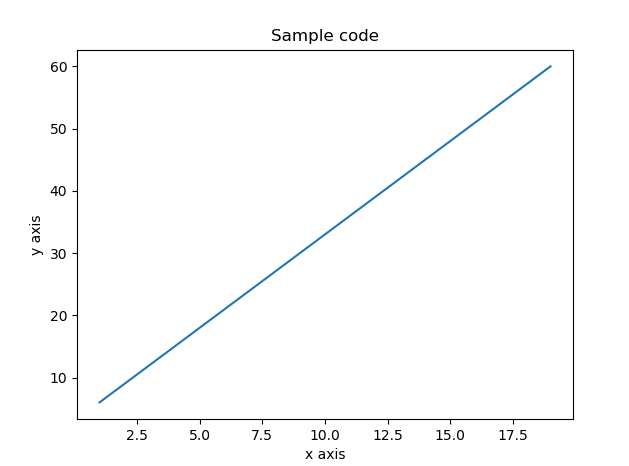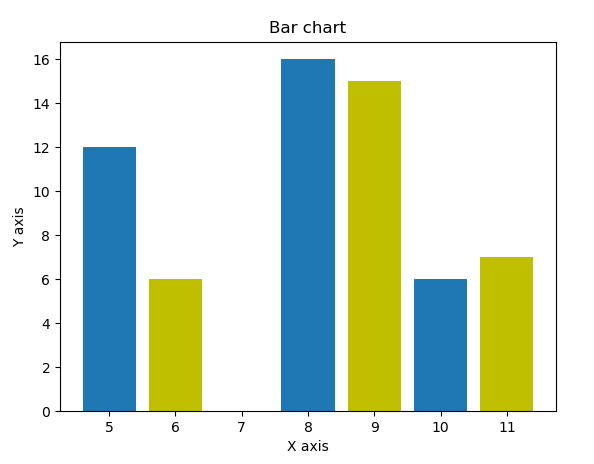# getting started with python numpy

Numpy is a python package which is used to perform a mathematical operation on the larger numeric dataset. We can also say that numpy is a multi-dimensional numeric array which is used to perform heavy mathematical operations.

Numpy array is mainly used with machine learning algorithms because in machine learning user needs to process larger data in real time. Rich and fast API numpy more popular for the machine learning tool

### Install Numpy

To install numpy use the below command in your terminal

\$ pip Install numpy

or

\$ pip install scipy

### Create a simple program using numpy

1. Create a file testnumpy.py
2. Open the file in your editor
3. Use the following code
4. In the below code we are using the one-dimensional array.

Example:

```import numpy as np
#create a 1 dimensional array in numpy
a= np.array([1,2,3])
print(a)

#Result = [1,2,3]```

### Attributes of numpy

Numpy provides a variety of attributes we will discuss them one by one.

Shape

the shape is mainly used for getting size of a numpy array and resize of numpy array

Example1: Get the shape of a numpy array

```import numpy as np
#create a 1 dimensional array in numpy
a= np.array([1,2,3])print("shape of array a {0}".format(a.shape))
array2= np.array([[4,5,6],[7,8,9]])
print("Shape of array2 {0}".format(array2.shape))

#Result = [1,2,3]```

Result:

shape of array a (3,)

Shape of array array2 (2, 3)

Reshape: we can resize the numpy array using the help of reshape attribute

Example2:

Reshape functionality

```import numpy as np
originalarray = np.array([[1,2,3],[4,5,6]])
print("size of original array {0}".format(originalarray.shape))
reshapedarray = originalarray.reshape(3,2)
print("size of resized array {0}".format(reshapedarray.shape))

###results

size of original array (2, 3)
size of resized array (3, 2)
```

itemSize: Itemsize is used to get the size of each element in an array.

Let’s understand it by below example

```import numpy as np
orignalarray = np.array([1,2,3,4,5,6,7,8,9],dtype=np.int8)
#get size of 1 dimensional array
print("Size for interget type {0}".format(orignalarray.itemsize))
twodarray = np.array([1,2,3,4,5,6,7,8,9],dtype=np.float32)
# we are now slicing array from index 1
print("size for float type {0}".format(twodarray.itemsize))

###results
Size for interget type 1
size for float type 4

```

Numpy also provide many more attributes

1. ndim
2. flag

Indexing and slicing

Extract data from numpy array is very easy and flexible. We can extract data like as we do in the simple array (BY index)

Let’s understand it step by step by some example

Suppose we have a numpy array like [1,2,3,4,5,6,7,8,9] if we want to extract item 4 then its index will be 3 because index start from 0

Example1:

```>>> import numpy as np
>>>
>>> orignalarray = np.array([1,2,3,4,5,6,7,8,9])
>>>
>>> # we are extracting an element from numpy array from index 3
... print("Element at index 3 is {0}".format(orignalarray))
Element at index 3 is 4
>>>
```

If we want to extract data for a specific range then we can define the range to it.
Example 2:
In the below example we are defining the starting index and ending index orignalarray[3:5] where 3 is starting index and 5 is ending index.

```>>> import numpy as np
>>>
>>> orignalarray = np.array([1,2,3,4,5,6,7,8,9])
>>>
>>> # we are extracting an element from numpy array from index 3
... print("Element in range from 3 to 6 is {0}".format(orignalarray[3:6]))
Element in range from 3 to 6 is [4 5 6]
>>>
```

Example3:
If we want to take all elements after a specific index then we need to provide stating index and then: e.g. [3:]

```>>> import numpy as np
>>> orignalarray = np.array([1,2,3,4,5,6,7,8,9])
>>> # we are extracting an element from numpy array from index 3
... print("Element in range from 3 is {0}".format(orignalarray[3:]))
Element in range from 3 is [4 5 6 7 8 9]
>>>```

#### How to do indexing on a multidimensional array?

```>>> import numpy as np
>>> # lets take we have a multidimensional array
... orignalarray = np.array([[1,2,3],[4,5,6],[7,8,9]])
>>> #get size of 1 dimensional array
... print("Array after slice {0}".format(orignalarray[1:]))
Array after slice [[4 5 6]
[7 8 9]]```

#### Math function on numpy array

Numpy provides Variety of math functions like trigonometric, arithmetic functions to handle complex calculations.
Let’s understand this by some examples
Numpy provide trigonometric functions like:

1. sin
2. cos
3. tan
Below example show how to use these functions:

```>>> import numpy as np
>>>
>>> array = np.array([0,30,45,90])
>>>
>>> # sin of following is
... print("sin of 0, 30, 45, 90 is {0}".format(np.sin(array*np.pi/180)))
sin of 0, 30, 45, 90 is [0.         0.5        0.70710678 1.        ]
>>>
>>> # cos of following is
... print("cos of 0, 30, 45, 90 is {0}".format(np.cos(array*np.pi/180)))
cos of 0, 30, 45, 90 is [1.00000000e+00 8.66025404e-01 7.07106781e-01 6.12323400e-17]
>>>
>>> # sin of following is
... print("tan of 0, 30, 45, 90 is {0}".format(np.tan(array*np.pi/180)))
tan of 0, 30, 45, 90 is [0.00000000e+00 5.77350269e-01 1.00000000e+00 1.63312394e+16]
>>>
```

Numpy also provide many different trigonometric functions like arcsin, arcos, arctann, round

Let’s take some more example
1. round
2. floor
3. ceil

```>>> import numpy as np
>>>
>>> array = np.array([1.99,55.789,77.44,88.99])
>>>
>>> print("Actual Array")
Actual Array
>>> print(array)
[ 1.99  55.789 77.44  88.99 ]
>>>
>>> print("After round to 2 decimal")
After round to 2 decimal
>>> print(np.around(array,decimals=2))
[ 1.99 55.79 77.44 88.99]
>>>
>>> print("After round to 1 decimal")
After round to 1 decimal
>>> print(np.around(array,decimals=1))
[ 2.  55.8 77.4 89. ]
>>>
>>> ## Lets calculate floor of a number
...
>>> print("floor of input array")
floor of input array
>>> print(np.around(array))
[ 2. 56. 77. 89.]
>>>
>>> ## Lets calculate floor of a number
...
>>> print("Celi of input array")
Celi of input array
>>> print(np.ceil(array))
[ 2. 56. 78. 89.]
```

#### Sorting, searching on numpy

Numpy provides a variety of searching and sorting function which is implemented on quicksort, mergesort and heapsort we can select which type of sorting we want to implement.
How to decide which type of sorting method we can use?
We can decide the it on the basis of space and time complexity. Below are the time complexity of all algorithms
1. quicksort O(n^2)
2. mergesort O(n*log(n))
3. heapsort o(n*log(n))
Above terms may be a little complex for you, but these are the way to  speed of a program.

Lets take some example.

```>>> import numpy as np
>>>
>>> ## Lets create a array with random function
... array = np.random.permutation(30)
>>>
>>> print("Actual Array")
Actual Array
>>> print(array)
[17 28  5  4  6 18 20 23 21 12 16 27  0 19  9 25 10  3  8  2 22 14 15 29
24  1 26 13  7 11]
>>>
>>> ## numpy sort
... print("After sort")
After sort
>>> print(np.sort(array))
[ 0  1  2  3  4  5  6  7  8  9 10 11 12 13 14 15 16 17 18 19 20 21 22 23
24 25 26 27 28 29]
```

Numpy provide a variety of other sorts
1. argsort()
2. lexsort()
3. argmax()
4. argmin()
you can review the same on official site of numpy which at bottom of the article.

#### Copy data in numpy matplotlib

Here we come with new term matplotlib

What is matplotlib?
Matplotlib is an open source python library which is used to draw data on chats.
Matplotlib provides a variety of chats, here we will use it with numpy.

Sample code:

```import numpy as np
from matplotlib import pyplot as plt

## Lets create a array with random function
x = np.arange(1,20)
y= 3*x+3

## Plt is ploat library
plt.title("Sample code")
# Defining x lable
plt.xlabel("x axis")
#defining y lable
plt.ylabel("y axis")
# ploating data
plt.plot(x,y)
#showing chart
plt.show()```

Result:We can draw different type of chart by using numpy like:
Sign chart, bar chart
Bar chart example:

```from matplotlib import pyplot as plt
corx = [5,8,10]
cory = [12,16,6]

corx2 = [6,9,11]
cory2 = [6,15,7]
plt.bar(corx, cory, align = 'center')
plt.bar(corx2, cory2, color = 'y', align = 'center')
plt.title('Bar chart')
plt.ylabel('Y axis')
plt.xlabel('X axis')

plt.show()```